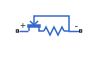# Current Limiter

Behavioral model of current limiter

•Libraries:
Simscape / Electrical / Semiconductors & Converters

## Description

The Current Limiter block provides a behavioral model of a current limiter. Use it to represent current limiting as found in power supplies and motor drives, and also to represent components that are used to limit inrush current.

The current limiting acts for both positive and negative currents. For applications where limiting is required in only one direction, you can augment the Current Limiter block with a series diode (blocks any reverse current) or parallel diode (no limiting in the reverse direction).

The block implements current limiting by using a hyperbolic tangent function:

`$i={i}_{LIM}\mathrm{tanh}\left(\frac{4v}{{v}_{LIM}}\right)+{g}_{LIM}v$`

where:

• i is the current through the component.

• v is the voltage drop across the component.

• iLIM is the current limit.

• vLIM is the approximate voltage drop across the component when the current limit becomes active.

• gLIM is the rate of change of current with voltage drop when on the current limit (limit-state conductance).

When v = vLIM, then

`$i={i}_{LIM}\mathrm{tanh}\left(4\right)+{g}_{LIM}v=0.9993{i}_{LIM}+{g}_{LIM}v$`

Therefore the current is approximately equal to the limit. Choose the value for gLIM such that gLIM·v is small compared to iLIM for the maximum expected voltage drop. This term is included in the block equation to improve numerical properties during simulation.

When choosing the value of vLIM, consider that making it too small will require tight solver tolerances and small step sizes. In practice, current limiters can be implemented using a MOSFET and series source resistor, the gate-source voltage being driven by the series resistor. This implementation does not produce a sharp limit, similar to the tanh curve used in this block. You can use a datasheet plot of current against voltage to pick a suitable value for vLIM.

### Thermal Port

This block has one optional thermal port. To expose the thermal port, set the Modeling option parameter to either:

• `No thermal port` — The block does not contain a thermal port.

• `Show thermal port` — The block contains one thermal conserving port.

The thermal port model contains a thermal mass. The power dissipated by the current limiter, plus the heat flow into the thermal port, drives the thermal mass differential equation:

`$m\frac{dT}{dt}={P}_{loss}+{Q}_{H}$`

where:

• m is the thermal mass.

• T is the thermal port temperature.

• Ploss is the electrical loss, v·i.

• QH is the heat flow from the external network into the thermal port.

## Ports

### Conserving

expand all

Electrical conserving port associated with the current limiter positive terminal

Electrical conserving port associated with the current limiter negative terminal

Thermal conserving port.

#### Dependencies

To enable this port, set Modeling option to `Show thermal port`.

## Parameters

expand all

Whether to enable the thermal port of the block and model thermal parameters.

### Parameters

The maximum current magnitude.

When the voltage drop is equal to this value, then the current is limited at 0.9993 times the current limit value.

When the current is limited, this parameter defines the rate of change of current with voltage drop if the current is driven harder onto the limit.

### Thermal Port

To enable these parameters, set Modeling option to `Show thermal port`.

The heat energy required to raise the temperature by one degree.

The temperature at the start of the simulation.

## Version History

Introduced in R2015a